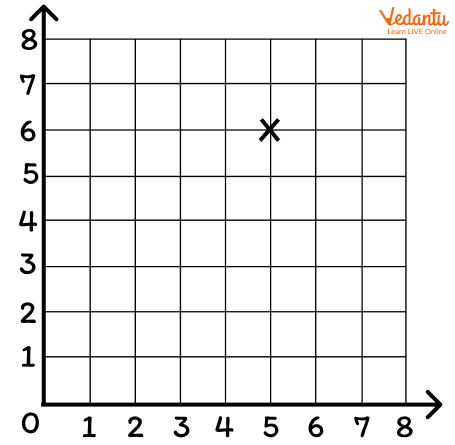Courses
Courses for Kids
Free study material
Free LIVE classes
More

# Teaching Shapes in Year 5## An Introduction to Shapes to Year 5 Kids

Kids are introduced to shapes and figures at an early age. Kids starting in Year 5 are introduced to the basic shapes and geometry. By Year 5, kids are familiarised with concepts like different shapes that they encounter every day. For example, we all have looked at and played with a ball, but have you wondered how we define its shape? The shape of a ball is a circle, similarly, a box is of a square shape. To learn more about shapes and how one can teach about shapes to Year 5 kids let us read the tips and tricks provided below.

We hope it would help you in teaching shapes to Year 5 kids, let us first discuss the concepts that can be taught to kids.

## What Are the Topics Covered in Shapes in Year 5?

Before discussing the teaching methods used to teach shapes in a fun way, let us look into the topics that are covered in the introduction of shape and geometry.

• The kids are taught to identify different shapes such as squares, rectangles and triangles based on their appearance.

• Kids are taught about the different types of angles. Examples of angles that are introduced include acute, obtuse, right and straight angles.

• Kids are also introduced to the use of protractors to measure the given angles.

• The properties of individual shapes are taught to kids in Year 5. This can include the properties of a rectangle. Rectangle characteristics will be familiar to the child. For example, kids will understand that all rectangles have two sets of parellel lines, four corners, and four right angles and that the angles in all rectangles total up to 360 degrees.

## How Can Parents Teach Kids Shapes in Year 5?

As we have discussed The topics that are taught under shapes in Year 5, let us now look into some of the fun cavities and methods that can help both parents and teachers in teaching shapes to kids in Year 5.

## Using Mathematical Terms While Practising

One may help children learn by assisting them in describing shapes using features such as:

• the total number of sides and edges

• the number of vertices/corners the number of faces

• lines of symmetry types of angles inside shapes.

Encourage children to use terms like diagonal, vertical, horizontal, parallel, acute, obtuse, and right angle in their Maths. The use of terms can help kids to better understand the meaning of the phrases.

## Use Protractor Mat with Kids

One of the most effective ways of teaching kids shapes in Year 5 is the use of protractors. As the instrument is used to measure the angles this activity can be both fun and beneficial. Make a 'protractor mat' for your children to practise using a protractor accurately. Create a 90-degree mat in the pattern of a quarter circle using the corner of a sheet of paper. For each 10°, draw a line. Alternatively, make a half-circle mat with an angle of 180 degrees. For each 10°, draw a line. This will assist them to become accustomed to the appearance of various degrees of increments.

## Playing Games That Require the Use of Coordinates

One of the games that require the use of coordinates is the game named battleships. Coordinates are integers that show where a point on a map, grid, or graph is located. Brackets are used to record coordinates, with the two numbers separated by a comma, as in: (1, 2). The first integer in a coordinate indicates the location of the point on the x-axis also called the horizontal axis) The second number indicates the location of the point on the vertical axis, often known as the y-axis.

Reading and plotting coordinates can help your youngster learn. The coordinates for the plotted point in the example below are (5, 6), where 5 is the x-axis value and 6 is the y-axis value:Graph representing the coordinates

In conclusion of the article, we have learnt about the topics that are usually taught in the introduction to shapes in Year 5. We have also learnt about some of the methods and activities that can be used in teaching shapes in Year 5.

Last updated date: 28th Sep 2023
Total views: 132k
Views today: 4.32k

## FAQs on Teaching Shapes in Year 5

1. Name some angles that can be taught to kids in Year 5.

Some of the angles that can be taught to kids include, acute, obtuse, right and straight angles. The angles that lie between 0 to 90 degrees are categorised as acute angles. The angle that lies between 90 to 180 degrees is said to be the obtuse angle, they are in a sense opposite to the acute angles. The right angle is defined as 90 degrees. 91 degrees is considered an obtuse angle whereas 89 degrees is considered to be an acute angle. Lastly, we can define the straight angle as an angle that is 180 degrees.

2. How to teach axes to kids?

Kids must first be introduced to coordinates in order to better understand the concepts of an axis. Coordinates are two numbers, or a letter and a number, that are used to pinpoint a specific point on a plane, also known as a coordinate plane. The x-axis, the horizontal line and the y-axis, the vertical line are the two axes in a coordinate plane. Axes refer to the "x" and "y" lines that form a graph when they cross at right angles.